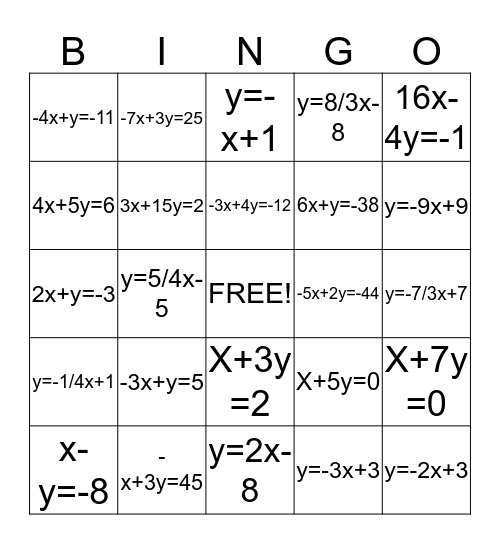# EquationsThis bingo card has a free space and 24 words: -3x+y=5, -x+3y=45, -3x+4y=-12, x-y=-8, 16x-4y=-1, -4x+y=-11, 4x+5y=6, -7x+3y=25, -5x+2y=-44, X+3y =2, 6x+y=-38, 2x+y=-3, X+5y=0, X+7y =0, 3x+15y=2, y=-3x+3, y=-7/3x+7, y=-x+1, y=-9x+9, y=5/4x-5, y=-2x+3, y=-1/4x+1, y=8/3x-8 and y=2x-8.

## Play Online